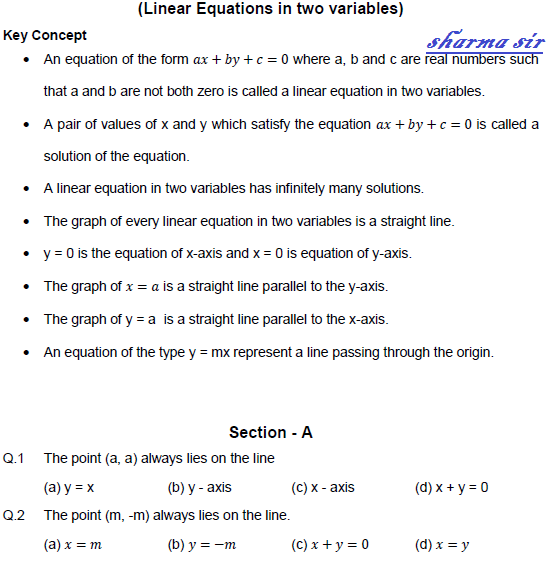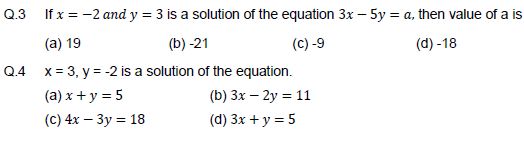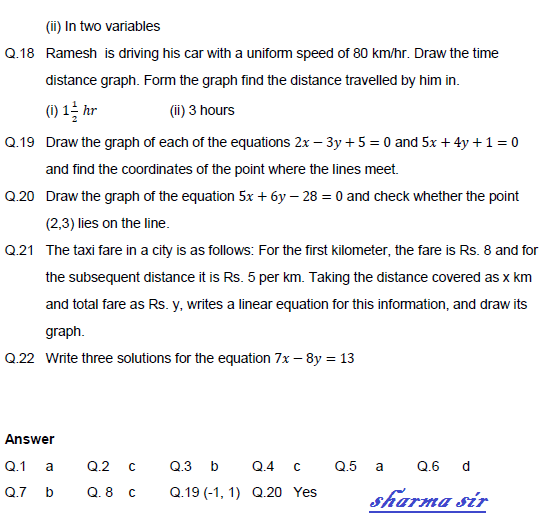# SCC Education

## Linear equations in two variable

Linear equations in two variableCircle-theorem-2

Circle-theorem-1

Theorems-of-triangles

Surface-area-and-volume

Herons-formula

Circle

Area-of-parallelograms

Elementry-algebra-questions

Polynomials

Formula-sheet-maths

Co ordinate geometry

Mensuration

Sample paper 2

Sample paper 3

Number system

Median of triangle

circle-theorem-2
circle-theorem-1
Theorems-of-triangles
important-rule-for-circle
surface-area-and-volume
herons-formula
circle
Area-of-parallelograms
elementry-algebra-questions
polynomials
formula-sheet-maths
co ordinate geometry

mensuration